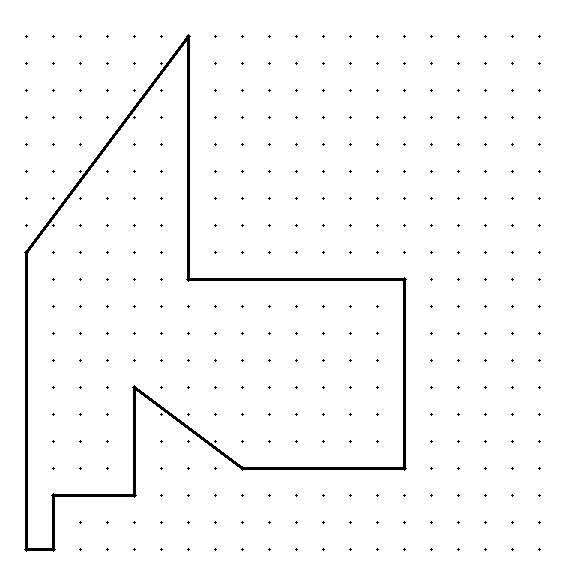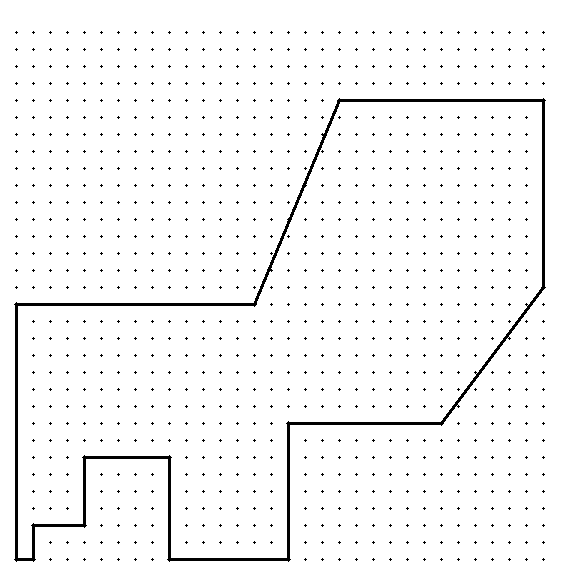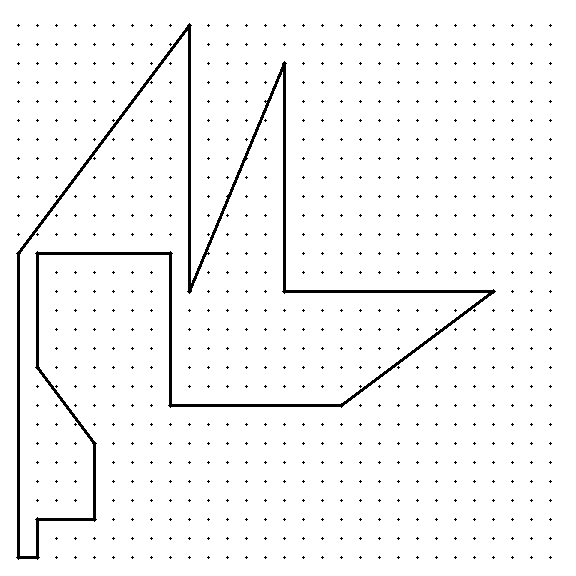# Lattice n-gons with ordered side lengths 1,2,3,…,n

Consider the octagon in the Cartesian plane with vertices at (0,0), (1,0), (1,2), (4,2), (4,6), (7,2), (7,8), and (0,8). Are there other (infinitely many) polygons, such as this, lying entirely in the first quadrant of the Cartesian plane with all its vertices at lattice points and whose sides, in anti-clock wise order, are precisely of lengths 1, 2, 3, ... n starting from the side departing at the origin?• I do not recall the references, but I do recall this problem being considered (perhaps even on MathOverflow), possibly without the prescription of the edges being in order. My feeling is that the obvious restrictions on horizontal and vertical lengths are the only ones (so that n satisfies some congruences mod 8 or mod 12 or something like that), and that such polygons exist otherwise. Gerhard "Sure I Saw It Before" Paseman, 2016.04.26. – Gerhard Paseman Apr 26 '16 at 15:29
• You may be referring to Martin Gardner´s "bicolor proof that every serial isogon of 90 degrees–a polygon with all right angles, and sides in 1, 2, 3, ... sequence–must have a number of sides that is a multiple of 8". – Bernardo Recamán Santos Apr 26 '16 at 15:34
• Thanks to Pythagoras sides need not be all horizontal or vertical. – Bernardo Recamán Santos Apr 26 '16 at 15:36
• You may be interested in golygons, which are orthogonal versions of your idea. See also MO question, "Can we find lattice polyhedra with faces of area 1,2,3,…?" for a 3D generalization. (This is likely what Gerhard remembers...) – Joseph O'Rourke Apr 26 '16 at 22:07
• So what is known about non-crossing golygons? The Wikipedia page only says "Finding the number of solutions that correspond to non-crossing golygons seems to be significantly more difficult." – domotorp Apr 29 '16 at 19:30

## 2 Answers

There are indeed other such polygons. -- For example there is one for $n = 11$, as follows (the origin is in the lower left corner):Also there is one for $n = 15$:Further there are $21$ such polygons for $n = 16$. One of them is the following:These polygons have been found with this GAP function.

There is one such polygon for $n = 8$, $n = 11$ and $n = 15$, each, there are $21$ such polygons for $n = 16$, and $225$ for $n = 19$. For all other $n < 20$ there are no such polygons.

The complete list for $n \leq 19$ in GAP-readable form can be found here.

Added on May 3, 2016: A zip file with *.png images of all $249$ polygons for $n \leq 19$ can be found here (545KB).

Added on May 4, 2016 (following a request in the comments): When leaving away the condition that the polygon is entirely in the first quadrant, we get $3$, $5$, $6$, $584$ and $882$ distinct polygons for $n = 8$, $n = 11$, $n = 12$, $n = 15$ and $n = 16$, respectively. A GAP-readable coordinate list can be found here, and a zip file with *.png images of all these $1480$ polygons can be found here (2.8MB).

• My conjecture is that there are infinitely many such polygons. Indeed, it could be that there is at least one with n sides for any sufficiently large n. – Bernardo Recamán Santos Apr 30 '16 at 17:41
• @BernardoRecamánSantos: It seems likely that your conjecture is true (e.g. further search found $225$ such polygons for $n = 19$). But Gerhard Paseman is right that $n$ must be congruent to $0$ or $3$ modulo $4$. -- Consider a checkerboard coloring of the integer points in the Cartesian plane. Then the ends of a side have the same color if its length is even, and they have different color if its length is odd. This holds also for diagonal sides, as you can check. So a closed path must contain an even number of sides of odd length. – Stefan Kohl Apr 30 '16 at 20:41
• Could you say something about how you searched for these (delightful) polygons? – Joseph O'Rourke Apr 30 '16 at 21:25
• @JosephO'Rourke: Of course! -- I have added a paragraph on this. – Stefan Kohl Apr 30 '16 at 22:31
• The number of all such serial polygons is now sequence A273089 in the OEIS: oeis.org/A273089. – Bernardo Recamán Santos May 16 '16 at 13:58

If one looks at "isopaths", which are paths starting from the origin, taking steps of lengths 1,2,3,... in that order and always landing on a lattice point, without regard to self crossing or staying in the first quadrant, one sees exponentially many isopaths in a polynomial area, so there will be exponentially many paths beginning and ending on the same point.

If we now observe directed isopaths, which are noncrossing and constrained to lie in the lower part of the first quadrant ( mostly on or below the line y=x, with exceptions made to accommodate the first few steps ), again we have for n sufficiently large exponential growth in n inside a polynomially bounded space. This is because for most n there will be two or more choices of how to proceed to avoid self intersection, even if no movement had been made toward the opposite quadrant (decreasing both x and y).

If we now observe directed isopath tails (including steps of length m greater than 1 up to n inclusive) the same reasoning again shows exponentially many isopaths which are non intersecting and limited to a polynomially sized region. We can take a location (x,x) which admits several isopaths to it of length m-1 "from below", and similarly find several isopath tails which return to the origin "from above", giving the desired polygon. One can ensure non-intersection by picking n large enough and asking for a path from (x,x) back to (0,n) using steps of length m up to n-1. Since diagonal lengths are allowed (and the displacement takes the form related to one like (2MN, M^2 -N^2) ), I see no parity or modular obstructions (Oops! now I see one; thus n has to be even to admit such a polygon) when n gets sufficiently large, so each (Oops again! each allowed) lattice point in a certain region should be the arrival point of many isopaths and isopath tails.

Gerhard "Confident There Are Infinitely Many" Paseman, 2016.04.26.

• This suggests the following approach to find numerical examples. For some small n, say n between 10 and 20, enumerate all directed isopaths, and then prune from that list all the spirals or other undesirable candidates that may not admit continuation. You should get a list of less than 20000 (x,y) pairs in the lower half of the quadrant which are endpoints of paths that stay within the lower part (or admissible part) of the rectangle between that point and the origin. Now grow this list by translation with n+1. Gerhard "Likes Ending Complex Problems Quickly" Paseman, 2016.04.26 – Gerhard Paseman Apr 26 '16 at 18:42
• Actually, a checkerboard coloring suggests for a cyclic isopath of n steps that n is 0 or 3 mod 4. Gerhard "Hoping For No More Restrictions" Paseman, 2016.04.26. – Gerhard Paseman Apr 26 '16 at 21:03